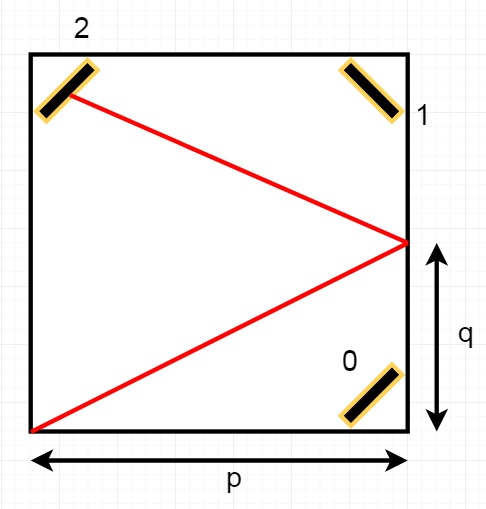# Mirror Reflection in C++

Suppose there is a special square room with mirrors on each of the four walls. In each corner except the southwest corner, there are receptors. These are numbered as 0, 1, and 2. Now the square room has walls of length p, and a laser ray from the southwest corner first meets the east wall at a distance q from the 0th receptor. We have to find the number of the receptor that the ray meets first.

So if p = 2, and q = 1, then the case will be like −So the output will be 2, as the ray meets receptor 2 the first time it gets reflected back to the left wall.

To solve this, we will follow these steps −

• while p and q both are even,
• p := p/2
• q := q / 2
• if p is even, then return 2
• if q is even, then return 0
• return 1.

Let us see the following implementation to get better understanding −

## Example

Live Demo

#include <bits/stdc++.h>
using namespace std;
class Solution {
public:
int mirrorReflection(int p, int q) {
while(p % 2 == 0 && q % 2 == 0){
p >>= 1;
q >>= 1;
}
if(p % 2 == 0) return 2;
if(q % 2 == 0) return 0;
return 1;
}
};
main(){
Solution ob;
cout << (ob.mirrorReflection(2, 1));
}

## Input

2
1

## Output

2